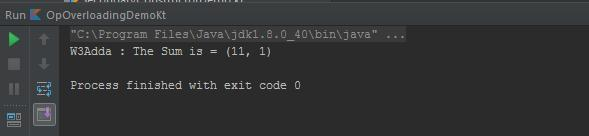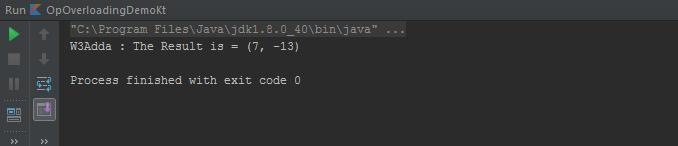In this tutorial you will learn about the Kotlin Operator Overloading and its application with practical example.

Kotlin allows us to provide implementation for predefined set of operators on our types. These operators have fixed procedure and fixed symbolic representation, like + or *. When you will use operator in kotlin so it’s corresponding member function is called.

Let’s see some operations.

Unary Operations:

 Expression Translated to +a a.unaryPlus() -a a.unaryMinus() !a a.not()

Increment and Decrement Operations:

 Expression Translated to a++ a.inc() a– a.dec()

Binary Operations:

 Expression Translated to a+b a.plus(b) a-b a.minus(b) a*b a.times(b) a/b a.div(b) a%b a.rem(b), a.mod(b) (deprecated) a..b a.rangeTo(b)

Example: Overloading + Operator: See below example which is using for plus operator.

Output: The output of this code will be like below image.In above example you can see that plus() is declared with operator keyword which will tell to complier that + operator will be overloaded. According to Above tables Expression point1+point2 will translated to point1.plus(point2).

Example: –Operator Overloading: –operator means decrement under the hood. In this example we will explain overloading of — operator. According to table suppose The expression is –a so it will translated to a.dec() for compiler and will tell that this operator is overloading.

Below line

is equivalent to

See below code.

Output: The output of above code will be.In this tutorial we have learn about the Kotlin Operator Overloading and its application with practical example. I hope you will like this tutorial.